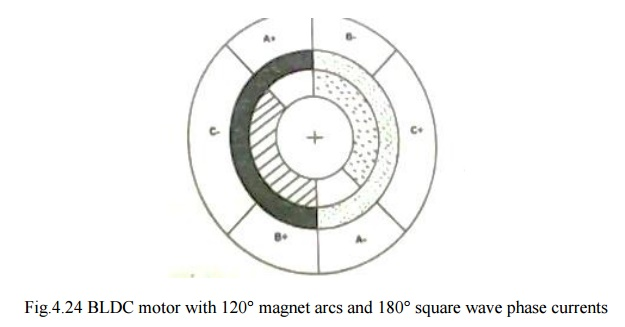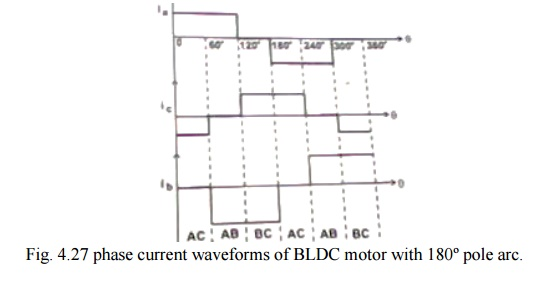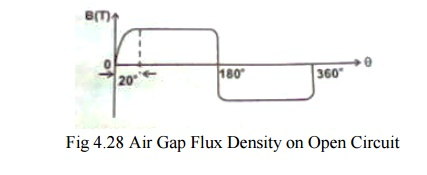Home | | Special Electrical Machines | Commutation in Motors With 120┬░ and 180┬░ Magnet Arc

# Commutation in Motors With 120┬░ and 180┬░ Magnet Arc

BLPM dc motor with 180┬░ magnet arcs and 120┬░ square wave phase currents arc shown in fig. 4.23 and 4.24.

COMMUTATION IN MOTORS WITH 120┬░ AND 180┬░ MAGNET ARC

BLPM dc motor with 180┬░ magnet arcs and 120┬░ square wave phase currents arc shown in fig. 4.23 and 4.24.In Fig. 4.26 the rotor magnet poles are shaded to distinguish north and south. The phase belts are shaded us complete 60┬░ sector of the stator bore. There are two slots in each of these phase belts. The current in these two slots are identical and conductors in them are in seriesBetween the rotor ring and the stationary belt ring in  fig. 4.26 there is a third ring  called the ŌĆ¢mmf ringŌĆ¢. This represents the mmf distribution of the stator currents at a particular instant.

v   At the instant shown wt=0, phase A is conducting positive current and phase C is conducting negative current. The resulting mmf distribution has the same shading as the N and S rotor poles to indicate the generation of torque,

v   Where the mmf distribution has like shasing, positive torque is produced. Where mmf and flux shading are unlike, negative torque is produced. Where one is zero, no torque is zero, no torque is produced. The total torque is the integral of the contributions from around the entire air gap periphery.

The rotor is rotating in the clockwise direction. After 60┬║ of rotation, the rotor poles start to ŌĆŚuncoverŌĆś the C phase belts and the torque contribution of phase C starts to decrease linearly.

During this period, the magnet poles, have been 'coveringŌĆś the B phase belts. Now if the negative current is commutated from C to B exactly at then point 60┬║, then the torque will be unaffected and will continue constant for a further 60┬║. After 120┬║, positive current must be commutated from A to C.

Commutation tables for three-phase brushless dc motors.

TABLE 4.1 180┬║ Magnet-Star Winding. 120┬║ Square wave phase Currentsv   The production of smooth, ripple free torque depends on the fact the magnet pole arc exceeds the mmf arc by 60┬║.

v   Here only 2/3 of the magnet and 2/3 of the stator conductors are active at any instantIn a practical motor the magnet flux-density distribution cannot be perfectly rectangular as shown in fig.4.27. for a highly coercive magnets and full 180┬║ magnet arcs there is a transition section of the order of 10-20┬║ in width. This is due to fringing effect. Likewise on the stator side, the mmf distribution is not rectangular but have a stepped wave form as shown in fig.4.28 that reflects the slotting.To some extent these effects cancel each other so that s that satisfactory results are obtained with a magnet arc as short as 150┬║, and two slots per pole per phase.

But there is always dip in the torque in the neighborhood of the commutation angles. This torque dip occurs every 60┬║ elec degrees, giving rise to a torque ripple component with a fundamental frequency equal to 6P times the rotation frequency where P is the number of pole pairs. The magnitude and width of the torque dip depends on the time taken to commutate the phase current.

Phase current waveforms corresponding to high speed and low speed operations are as shown in fig. 4.29 (a & b)(a)  High speed, full voltage. Note the dip caused by commutation of other 2 phases,

(b) Low speed with current controlled by chopping.

Fig.4.29 Phase current wave forms.

v   The back emf is of equal value in the incoming phase and is in such a direction as to oppose the current build up.

v   While the flux distribution of the magnet rotates in a continuous fashion, the mmf distribution of the stator remains stationary for 60┬║ and then jumps to a position 60┬║ ahead.

Similar analysis is made with a motor having 120 ┬║ pole arc magnets with delta connected armature winding.

Table 4.2 120┬║ Magnet Delta Winding, 180┬║ Square Wave Phase Currents.v   C phase belt remains covered by the magnet poles. While the coverage of A phase belt increases thereby decreasing that of B phase belt.

v   Since all the conductors are varying same current the increasing torque contribution of phase A is balancing by the decreasing contribution of phase B. Therefore, the total torque remains constant.

v   Similarly there is a linear increase in the back emf of A and equal and oppoaite decrease in the back emf in phase B, Therefore the back emf at the terminals remains constant.

v   Line current divides equally between two paths One-phase C Second-phase A & B series.

This balance is not perfect in practice because of the resistance and inductance of the windings.But the current balance should be maintained, otherwise circulating current may produce excessive torque ripple and additional losses.

When compared with 180┬░ pole arc machine.

v   For the same ampere-conductors per slot and for the same peak flux density, the 120┬░ pole arc machine has 1.5 times copper losses, but produces the same torque.

v   Also the ampere-conductors per slot would have to be reduced because the duty cycle is 1.0 instead of 2/3.

Merits

v   For the same magnet flux density the total flux is only 2/3 of that of 180┬░ pole arc motor, so that only 2/3 of the stator yoke thickness is required. If the stator outside diameter is kept the same, the slots can be made deeper so that the loss of ampere conductors can be at least partially covered .consequently the efficiency of the motor may not be very much less than that of 180┬░ pole arc machine.

v   In this machine also, the effects of fringing flux, slotting and communication overlap combine to produce torque ripple.

v   Only emf and torque are discussed. The concept of hanging flux-linkage and energy balance can also be used to analyze the operation.

Study Material, Lecturing Notes, Assignment, Reference, Wiki description explanation, brief detail
Special Electrical Machines : Permanent Magnet Brushless D.C. Motors : Commutation in Motors With 120┬░ and 180┬░ Magnet Arc |